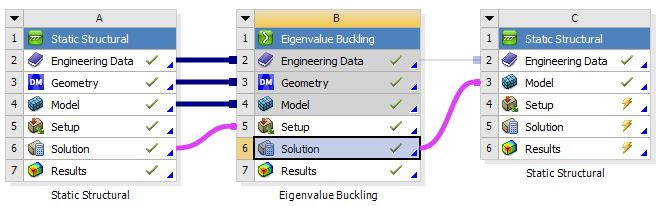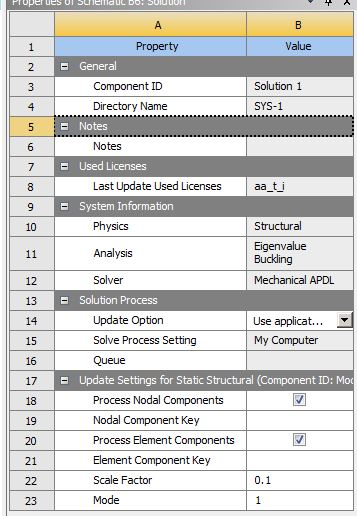## General Mechanical

•Venugopalb
Subscriber

Hello all!

I have done a linear buckling analysis in Ansys workbench. My ultimate aim is to calculate the critical buckling load of a structure with the inclusion of material and Geometric nonlinearity. However, I added material nonlinearity by feeding plasticity data of material. Now I need to add geometric imperfection.

Someone said Linear buckling analysis deformation scale factor can be used as a geometric nonlinearity. Is it correct? because I added it to the model. But it does not have any considerable amount of change in buckling load.Thanking you

•peteroznewman
Subscriber

It doesn't look like you have solved system C, the nonlinear Static Structural model. How did you determine the nonlinear buckling load from system C?

•Venugopalb
Subscriber

Hi Peter!

I used the ARCLEN method to solve the model. It does not solve the problem due to some distortion error. However, by using time increment we can calculate the applied load(critical buckling is a peak value we got before it drops down).

my question Can we use the deformation scale factor of linear buckling for geometric nonlinearity of the model?